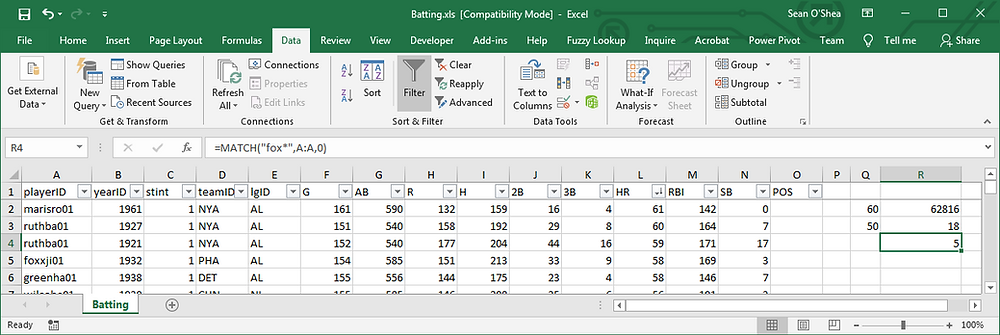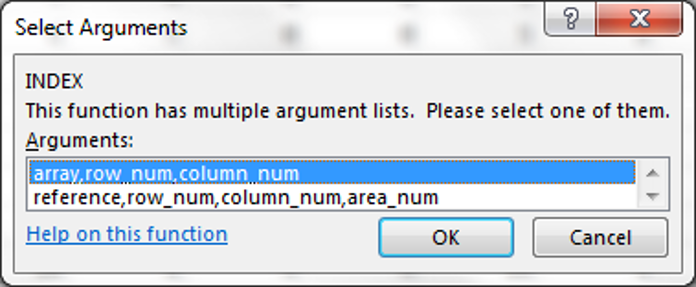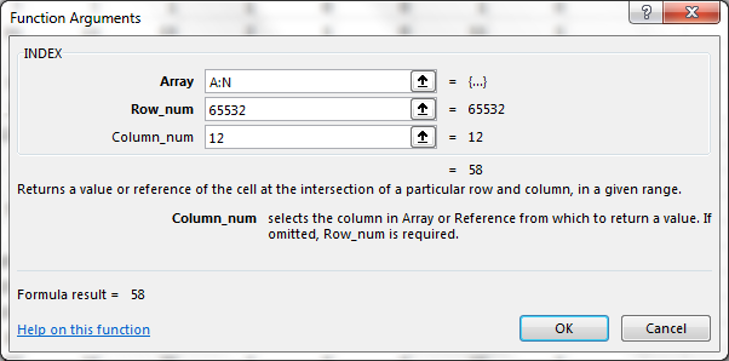Search

# INDEX and MATCH

Here's a refresher course on how the INDEX and MATCH formulas work in Excel.

If you insert the MATCH formula at Formulas . . . Lookup & Reference. . . you'll be given the option to:

1. lookup a value

2. in a selected array

3. according to a match type -

0 for an extact match

1 for the largest value that is less than or equal to the lookup value.

-1 for the smallest value that is greater than or equal to the lookup value.When the match type is 1 the array must be in ascending order.

When the match type is 0 the array must be in descending order.

In this example we see that the formula:

=MATCH(Q2,L:L,1). . . returns the row number of the first entry in column L that is equal to or greater than the value Q2. Note that the HR column is sorted in ascending order. If it is sorted in the opposite order the value will not change, and will be incorrect.

With the HR column sorted in descending order we can use the formula:

=MATCH(Q3,L:L,-1)

. . . to find the row on which the lowest number of home runs equal to or greater than the value in Q3 appears. So this data (which only goes through the 1988 MLB season) lists 17 players who have hit 50 or more home runs in a season.Wildcard characters (* for multiple characters; and ? for single characters) can be used with the MATCH formula - but only when the lookup value is 0. See for example, =MATCH("fox*",A:A,0)MATCH formula are usually nested inside INDEX formulas. See the Tip of the NIght for August 17, 2015. Knowing the row number where a value appears is not as helpful as knowing which value appears on that row. So if we edit the first formula to be:

=INDEX(A:A,MATCH(Q2,L:L,1))

. . . we get the name of the player who hit the highest number of home runs less than or equal to 60.When entering an INDEX formula from the 'Lookup & Reference' menu note that you'll have the option to structure the formula in one of two ways:You can list an array, then a row number and column number. . . to get this formula: =INDEX(A:L,65532,12). Here it is used to simply pinpoint a particular spot in an array.

The second option (for reference, row_num, column_num, area_num) will give the same result if the area_num is left blank. So this entry:. . . generates the same formula: =INDEX(A:N,65532,12) and result, 58.

The area_num gives the option of searching through in multiple arrays and then specifying which one a value should be returned from. So in this example:The formula =INDEX((H1:H3, J1:J3), 3, 1, 2) is returning the third row and first column of the second array.

We can also enter values directly in an INDEX formula. For example: